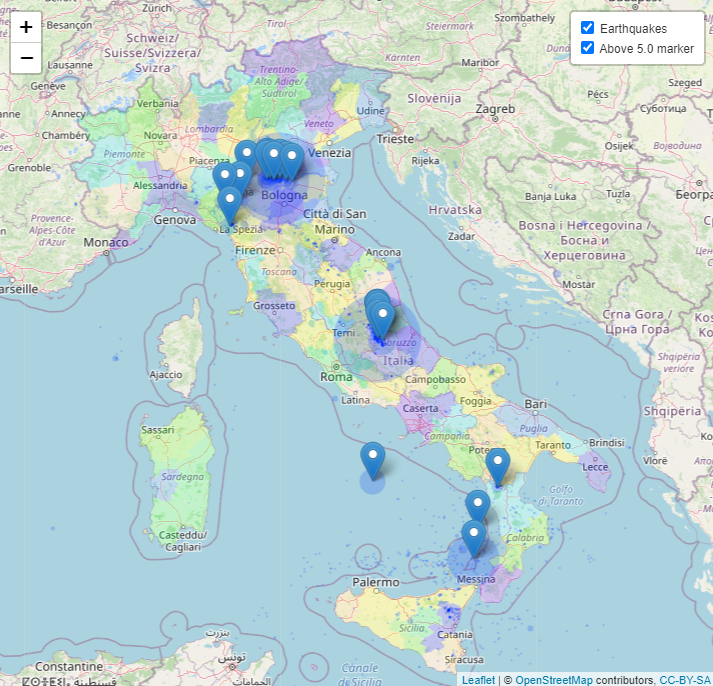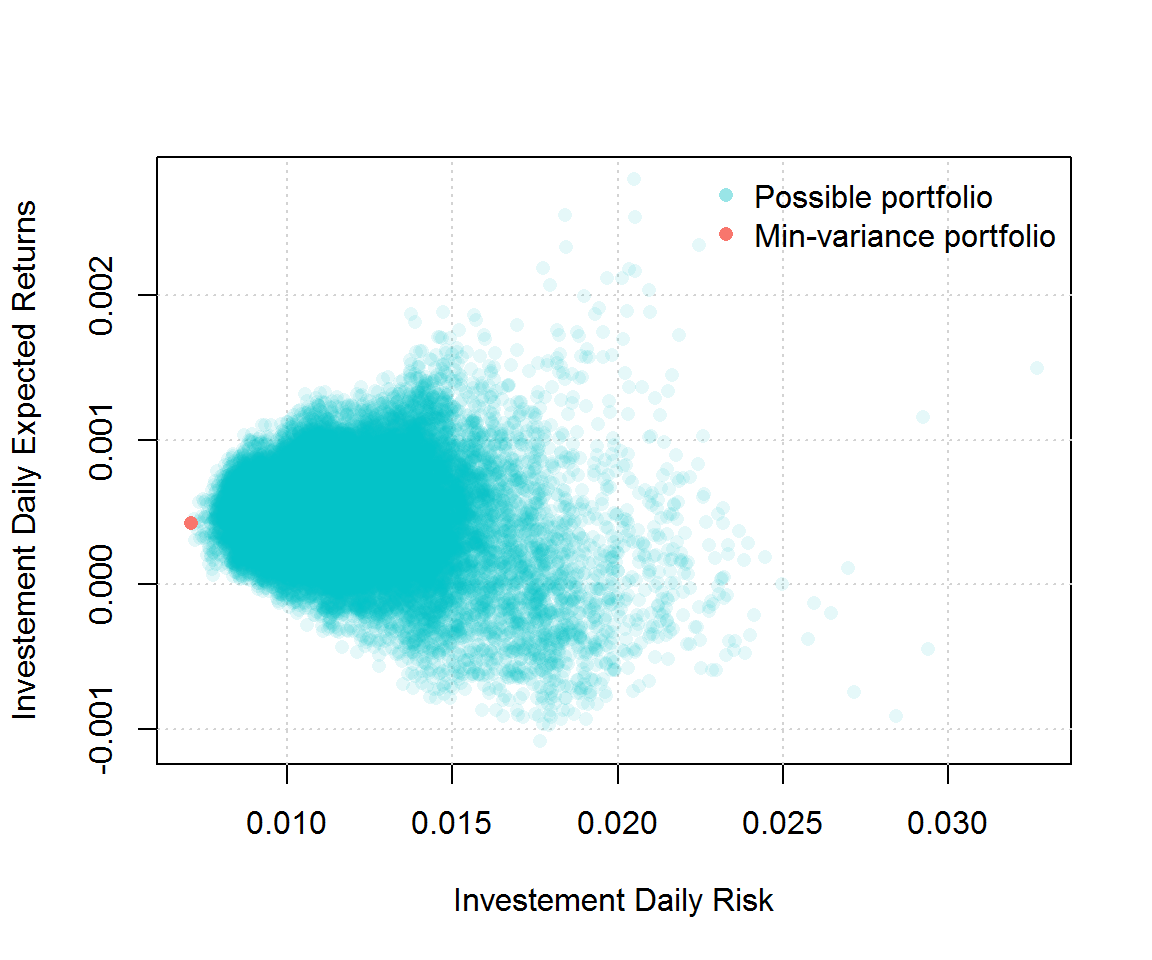# homework #2

The objectives of this homework assignment are the followings:

• Learn how program effectively using if/else and iterations statements;
• Become familiar with using data frame objects and mapping packages;
• Constructing a portfolio;
• Become familiar with GitHub and use it as a collaborative tool.

This project must be done using GitHub and respect the following requirements:

• All members of the group must commit at least once.
• All commit messages must be reasonably clear and meaningful.
• Your GitHub repository must include at least one issue containing some form of TO DO list.
• Organization (separation of work,…) and progress for your group must appear clearly in GitHub Projects.

You can create one or several RMarkdown files to answer the following problems:

#### Problem 1: Are you a power?

Write a program that prints the numbers from 1 to 100, but with the specific requirement:

• if the number is a full square of another number $a$, print a^2;
• if the number is a full cube of another number $b$, print b^3;
• if the number is a full square of another number $a$ and a full cube of another number $b$, print a^2, b^3.

An example of the output would be:

"1^2, 1^3" "2" "3" "2^2" "5" "6" "7" "2^3" "3^2" "10" "11" "12" "13" "14" "15" "4^2" "17" "18", ...

#### Problem 2: Map

This exercise is designed as a guided tutorial to the basic functions of the leaflet and maps packages that allow to draw maps and display information on them. First of all, if not done already, install leaflet and maps and load them into R.

• (a) Create a simple map by using the addTiles() function with its first argument being leaflet() and display the resulting map.
• (b1) On the map from point (a), add a marker with the help of the addMarkers function at $(\text{lon}, \text{lat})=(6.581188,46.522451)$ with a popup message specifying “University of Lausanne”.
• (b2) On the same map display one favorite place in Switzerland for each member of your team. To do so, create a data.frame (or tibble), find all corresponding coordinates, add the names of the places, add use addMarkers to the result of (b1).
• (c) Using the map function from the maps package, display a map of Italy with different colors for the various regions in this country, using the addPolygons function. See color options for filling the polygons.
• (d) Download and load the ETAS package in order to retrieve some earthquakes data for Italy, that are stored in the aforementioned package as italy.quakes (assign it to a variable into your R). Filter for earthquakes of magnitude greater than (or equal to) 4.0 on the Richter scale and add markers with popups on the various localization of these earthquakes with their respective magnitudes.
• (e) On the previous map in point (d), instead of simple markers, add circle for each of the earthquake using the addCircles function and let the size of the circle vary with the magnitude of the earthquake. Add markers for the earthquakes that have more or equal than 5.0 magnitude with a popup of the corresponding number. Finally, use addLayersControl to distinguish on the map between two types of datatips (groups): the circles of all earthquakes, and the markers of the earthquakes of a magnitude 5.0 or higher, using the overlayGroups parameter. Your final map should look similar to the following:#### Problem 3: Stock price and European call option

Let a stock price at time $t$ be $S(t) \equiv S_t$. Assume that $S(t)$ follows a Geometric Brownian Motion (GBM) given by:

$$\mathrm{d}S_t = r\,S_t + \sigma \, S_t \, \mathrm{d}W_t, \quad 0 < t < 1,$$

where $S(0) = 1$, $r$ is a percentage drift, $\sigma$ is a percentage volatility, and $W_t$ is a Brownian motion.

To simulate from this continuous time series model one can use the Euler-Maruyama discretisation method with a constant temporal step $\tau$:

$$S_{m+1} = S_m + r\,S_m\,\tau + \sigma\,S_m\,\Delta W_m, \quad S_0 = 1,$$

where $\Delta W_m$ are i.i.d. centered normally distributed random variables with variance $\tau$, $m = 0, 1, 2, \ldots, N_t - 1$, $N_t = \text{ceiling}(1/\tau) + 1$ is the number of temporal steps at which the values $S_m$ are calculated (including $0$ and the first temporal step that exceeds $1$, if necessary).

A European call option with the strike price $K$ can be expressed as

$$Y = \exp(-r) \cdot \max\{0; S(1) - K\}.$$

The value of $Y$ can be approximated using a Monte-Carlo method: consider $N$ Euler-Maruyama simulations of the paths $S(t)$; for each simulation $n = 1, 2, \ldots, N$ calculate $Y _{n}$ using the above mentioned formula; eventually, approximate the value of $Y$ by taking the mean $\bar{Y} = \sum _{n=1}^{N} Y _{n}$.

For the following parameters

Parameter Value
$r$ 0.05
$\sigma$ 0.2
$\tau$ 0.001
$K$ 1
$N$ 500
• (a) Simulate $N$ paths of the stock price $S(t)$ using the Euler-Maruyama method. Plot some of these paths and comment on them. Do you think this model reflect what you could observe on the stock market? What features seem striking?

• (b) Calculate $N$ European call option prices $Y_n$, $n = 1, 2, \ldots, N$ and plot the average option price Y_bar = mean(Y_n). Is it what you were expecting?

• (c) Find and plot with a green color the path $S(t)$ versus the time $t$, which led to the highest value of the option price, i.e. the path $S(t)$ corresponding to $\max(Y_n)$;

• (d) On the same graph plot the path $S(t)$, which led to the lowest value of the option price, i.e. the path $S(t)$ corresponding to $\min(Y_n)$;

• (e) Finally, on the same graph plot the path $S(t)$, which led to the value $Y_k$ that is the closest to the estimate $\bar{Y}$, i.e. the path $S(t)$ corresponding to $\min(|Y_n - \bar{Y}|)$.

#### Problem 4: portfolio construction

Suppose that you are working in an investment firm company as a quantitative analyst. Your boss gives you the task of creating a portfolio for one of your client. The client wants to find the portfolio with the smallest variance that satisfies the following constraints:

• Invest exactly \$1,000,000.
• Invest in exactly two stocks of the S&P500 index.

Therefore, your boss requires that you compute all possible portfolios that satisfy the client’s constraints, represent them graphically as (for example) in the graph below and find the weight of the best (i.e. minimum variance) portfolio.In order to complete this task, the boss tells you to use 3 years of historical data. The boss also mentions that the functions get() and ClCl() could be useful for this project and provides you with the example below (what a really nice boss!):

library(quantmod)
library(rvest)

sp500 %>%
html_nodes(".text") %>% # "td:nth-child(1) .text" should be used instead
html_text() -> ticker_sp500

SP500_symbol <- ticker_sp500[(1:499)*2+1]

# Replace "." by "-"
SP500_symbol <- gsub(".","-",SP500_symbol,fixed=T)

# Specify timing
tot_length <- 3 * 365
today <- Sys.Date()
seq_three_years <- seq(today,by=-1,length.out=tot_length)
three_year_ago <- seq_three_years[tot_length]

# Retrieve data for a stock
i <- 1
getSymbols(SP500_symbol[i],from=three_year_ago,to=today)
stock_price <- ClCl(get(SP500_symbol[i]))